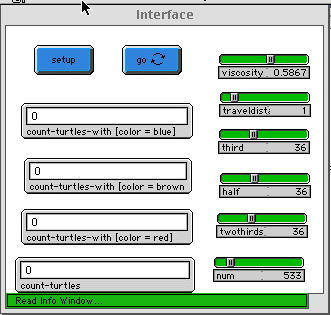WHAT IS IT ----------

This is an evolutionary game-playing model based on William Harms's "Divide the
Cake Model" from Brian Skyrms's "The Evolution of the Social Contract."  In this
model, there are three types of agents who must divide a common resource.  Only
two agents are permitted on any patch at any time.  Each of the agent's tries to
eat a certain amount of the patch's resources.  There are one-half agents,
one-third agents, and two-thirds agents, each of whom try to take the respective
amount of the patch's resources. If the total amount requested by both agents is
greater than 100%, then both agents die.  Otherwise, each agent gets his
requested share of the patch's resources.   Each agent then enters a reproduction
lottery based on its appetite:  the greater appetite, the greater the chance of
reproduction.  This factor gives a fitness advantage to the agents with a greater
appetite that counteracts the disadvantage of having a greater appetite (viz.,
the higher chance of asking for too much food and dying).  Each turn, every patch
resets to the full amount of food.

HOW TO USE IT -------------

SETUP:  Initializes the model to the values of the sliders, and creates the
agents.

GO:  Starts the model.

THIRD:  This slider sets the ratio of one-third agents.

HALF:  This slider sets the ratio of one-hald agents.

TWOTHIRDS:  This slider sets the ratio of two-thirds agents. Note:  as long as
the values of THIRD, HALF, and TWO-THIRDS are equal, the actual number chosen is
irrelevant.

NUM:  Creates this number of turtles

TRAVELDISTANCE:  The value of this variable determines the number of steps an
agent moves each turn.  This value is the mobility of an agent.

VISCOSITY:  This variable is the difficulty of movement.  It limits the general
mobility of agents.

THINGS TO NOTICE -----------------

If you run the model with the default settings (high viscosity), notice how the
half population increases.  Why does this happen?  Also, notice how the third and
two-thirds populations group onto each other.  Why does this happen?

The population increases very fast in the model, so the model is written stops
automatically when the population reaches a maximum threshold.  Does this affect
the model?

THINGS TO TRY -------------

Try changing the population VISCOSITY value.  What happens when this value is
decreased?  How does this affect the survival and the grouping of the different
populations?

Change the starting ratios of the populations.  How do these ratios affect the
behavior of the model?  Why should the model depend on these ratios?

Change TRAVELDISTANCE.  How does it affect the model?  What is the relationship
between the values of TRAVELDISTANCE and VISCOSITY?

EXTENDING THE MODEL -------------------

What environmental variables might affect the model?  Should the grass grow back
to its full length each turn?  How would changing the available resources affect
the model?

Should the turtles be able to ask each other what they are going to ask for
before asking?  If they did, then turtles could bargain before asking for food,
and therefore reduce their chances of dying by asking for too much.  This
bargaining behavior changes the model drastically, but is an interesting way of
exploring how populations might stabilize.

RELATED MODELS --------------

Altruism (Trait) Model Cooperation (Behavior) Model